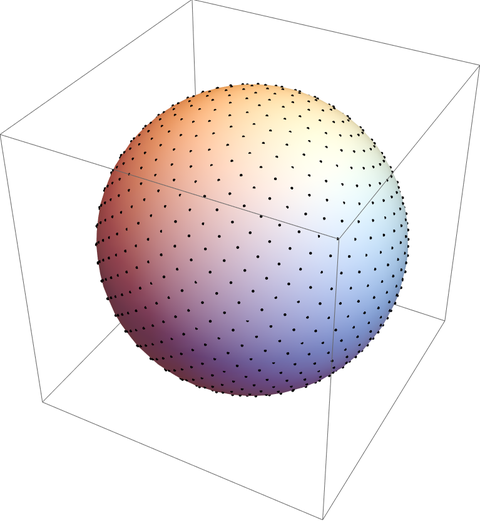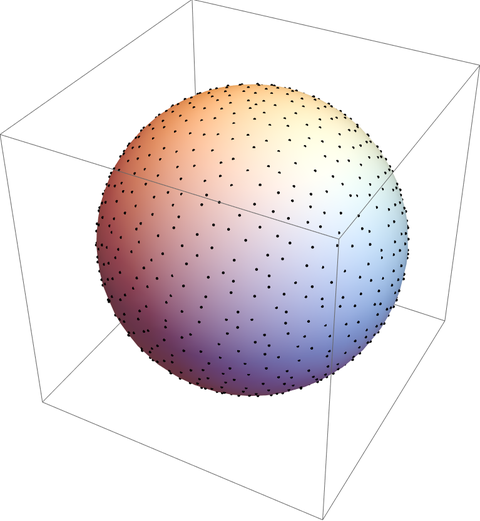# Simple way to distribute points on a sphere

Evenly placing points on a sphere is a difficult problem. It’s impossible in general, and so you distribute the points as evenly as you can. The results vary according to how you measure how evenly the points are spread.

However, there is a fast and simple way to distribute points that may be good enough, depending on your application, called the Fibonacci lattice.

Let P = 2N + 1. To distribute P points on a sphere let the latitude of the points be arccos(2i/P) and the longitude 2πi/φ where φ is the golden ratio and i runs from −N to N.Here’s the Mathematica code that produced the image above.

```    sp[{r_, lat_, long_}] := r {Cos[lat] Cos[long], Cos[lat] Sin[long], Sin[lat]};
n = 500;
Graphics3D@{
Sphere[{0, 0, 0}, 1],
Point[sp /@
Table[{1, ArcSin[2 i /(2 n + 1)], 2 Pi i/GoldenRatio},
{i, -n, n}]]}
```

Maybe you’d like something that looks more random, i.e. something that looks what people think randomness looks like. Actual randomness is clumpier than people expect. In this case you could jitter the Fibonacci lattice by changing the coordinates to something like

`    {1, ArcSin[2 i /(2 n + 1)] + RandomReal[0.05], 2 Pi i/GoldenRatio + RandomReal[0.1]}`

Note that this adds twice as much randomness to the longitude than latitude because the former has twice the range.